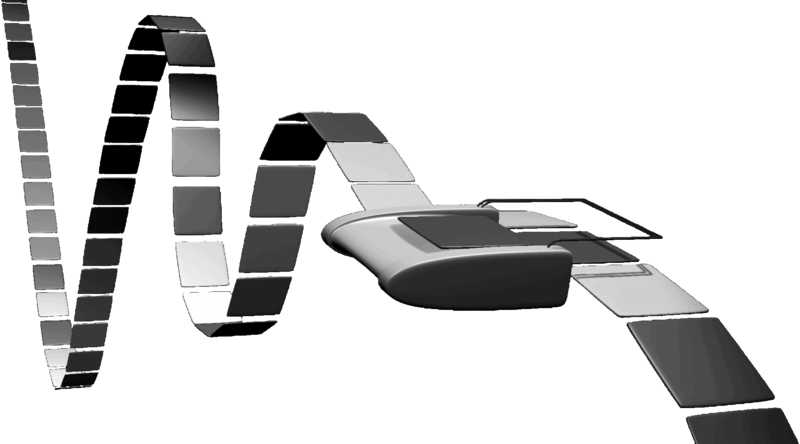And here we are with what I think is the most important “new” concept in this course: the Turing Machine. Roughly speaking, these are automata that are equivalent in power to what we think of normal computers today. Therefore, any problems that are unsolvable by Turing Machines are beyond the limit of current computation. Let’s briefly go over the automata we’ve seen thus far:

1. Finite Automata (DFAs and NFAs)
2. Pushdown Automata (PDAs and NPDAs)
3. Turing Machines (TMs, NTMs)

The N’s denote the nondeterministic versions. In other words, they allow multiple branches of computation to proceed simultaneously, rather than having one strictly defined path for each input string as would be the case with deterministic automata.

Here, the automata are listed in order of increasing power. Finite automata recognize the class of regular languages. Pushdown automata recognize the class of context-free languages. All context-free languages are regular, but not all regular languages are context-free. This is why pushdown automata are considered more “powerful” than finite automata, with respect to computability. Their infinite stack gives them more memory that can be useful to solve certain non-regular languages.

But Turing Machines (abbreviated as TMs), which recognize the class of decidable and recognizable languages (to be explained in a future blog entry), take things a step further. Like finite automata and pushdown automata, TMs have states and use transition functions to determine the correct path through states to take upon reading in an input string. But they have an additional feature called an infinite tape that is depicted in the picture above. There is also a tape head that reads in one symbol from a TM. This is a fairly abstract concept, and it’s often surprising to first realize that this simple addition to an automata allows it to recognize the class of all computable functions.

For instance, if we wanted to compute the value of a function, such as a function that returns a value twice as much as its input $f(x) = 2x$, then assuming our alphabet consists of 0s and 1s, the infinite tape starts with the binary symbol for the input, and then once the computation is over, contains the binary symbol for the output. Thus, the infinite tape can be the medium for which input/output occurs. Obviously, a TM must have some way of modifying the tape, and there are two ways of doing so.

1. The function $\delta(q, a, L) = (q', b)$ means that, if at state $q$ and the head reading in an $a$, the TM will replace the $a$ with a $b$ on the tape, move to state $q$, and move the tape head left.
2. The function $\delta(q, a, R) = (q', b)$ means that, if at state $q$ and the head reading in an $a$, the TM will replace the $a$ with a $b$ on the tape, move to state $q$, and move the tape head right.

That’s literally all we need to know about a TM’s transition function. That the tape head can move along the infinite tape and replace symbols on the tape from anything in the TM’s pre-defined “tape alphabet” is what allows a TM to go through a computation. There’s a small caveat: we obviously need a place for the tape head to start out on the tape! So when we say the TM’s tape is infinite, we really mean it’s infinite in the rightward direction. That is, there is a “bumper” that indicates the start of the tape, and the rest of the tape extends to the right. Therefore, if the TM’s tape head is on the first symbol of the tape, it can’t move left since the bumper prevents it from doing so. Thus, having a transition function that causes the tape head to move left while at the leftmost symbol of the tape will just leave the tape head where it is.

Another important thing to know about the tape is that, since it’s infinite, we need to have a symbol on each component. The input string takes up the first few segments of the tape, but beyond that, the tape is composed of what’s known as the blank symbol. These are part of the tape alphabet, so it’s legal to put them on the tape, as well as replace them if necessary.

The infinite tape is not the only feature that makes TMs different from finite automata (for instance, TM’s have only one accept and one reject state, and their effects take place immediately), but it’s by far the most important one. To understand a TM, it is necessary to have a “good feel” of how the tape works in your head. I can’t emphasize this enough. Don’t waste time trying to get the technical details of the Turing Machine. Use a high-level view of them. Make sure you get an intuitive understanding of how they work. I like to do this by imagining arbitrary computation paths and moving the tape head around the infinite tape.

Let’s do a quick example question that highlights the importance of understanding how a TM works over the nitty gritty details.